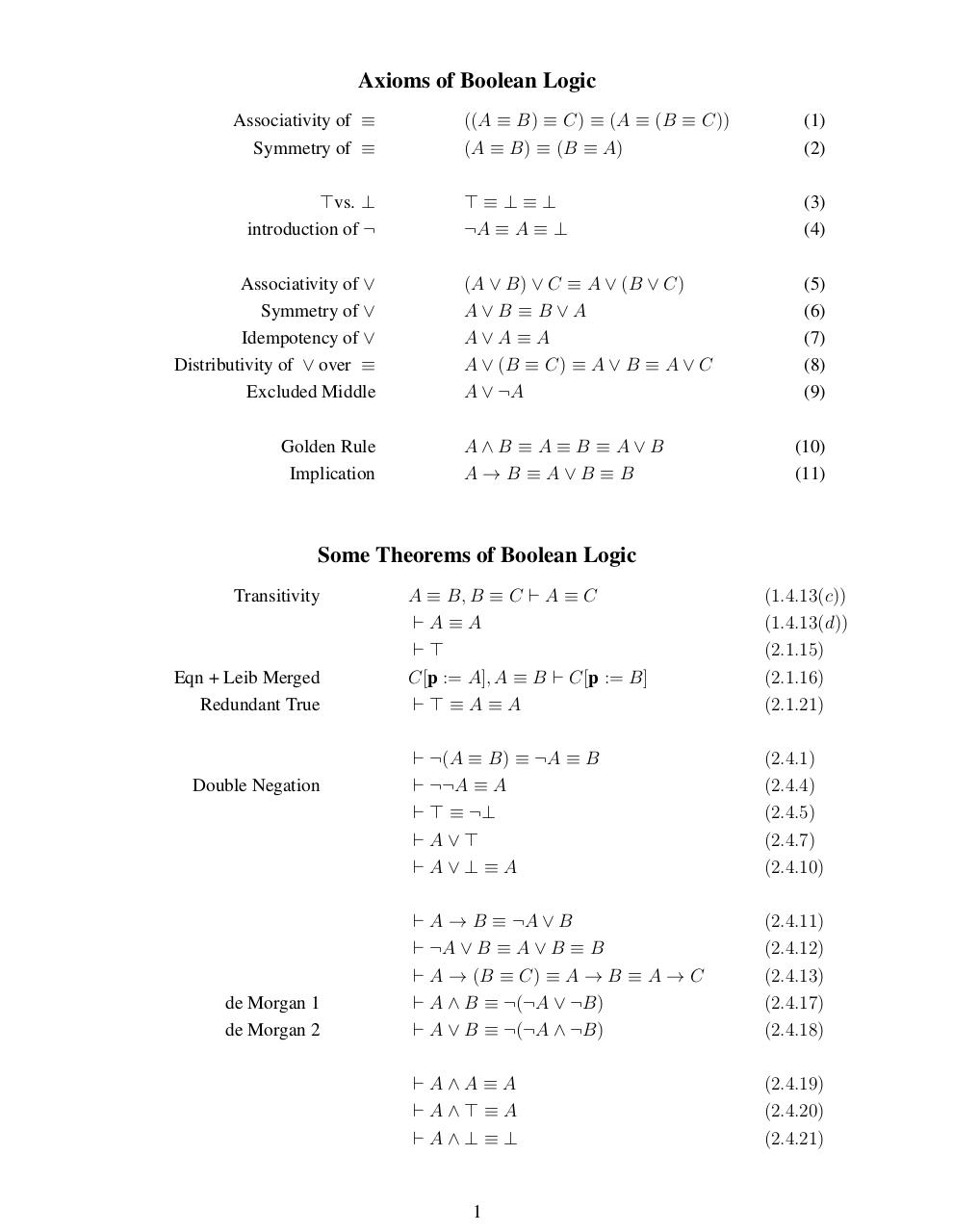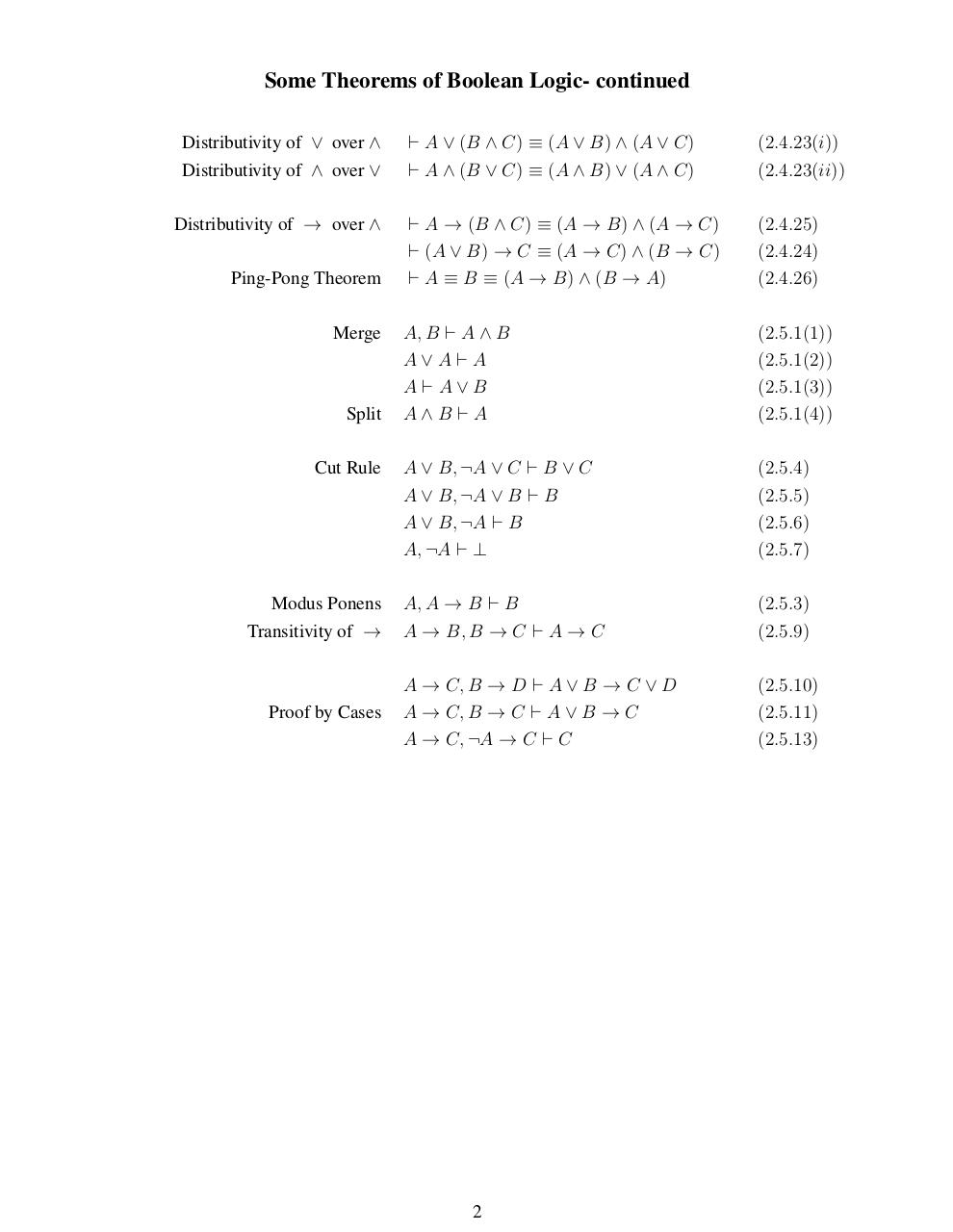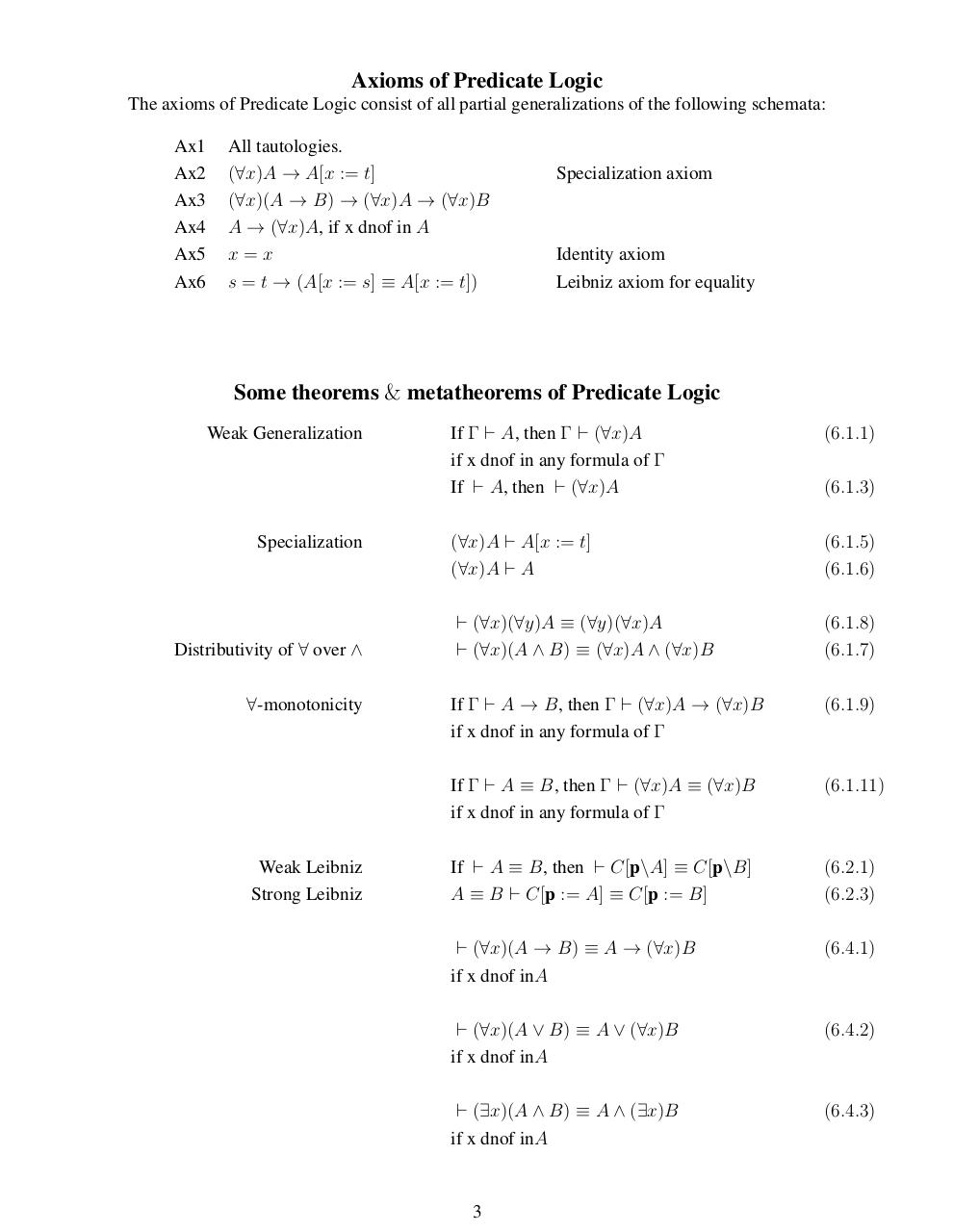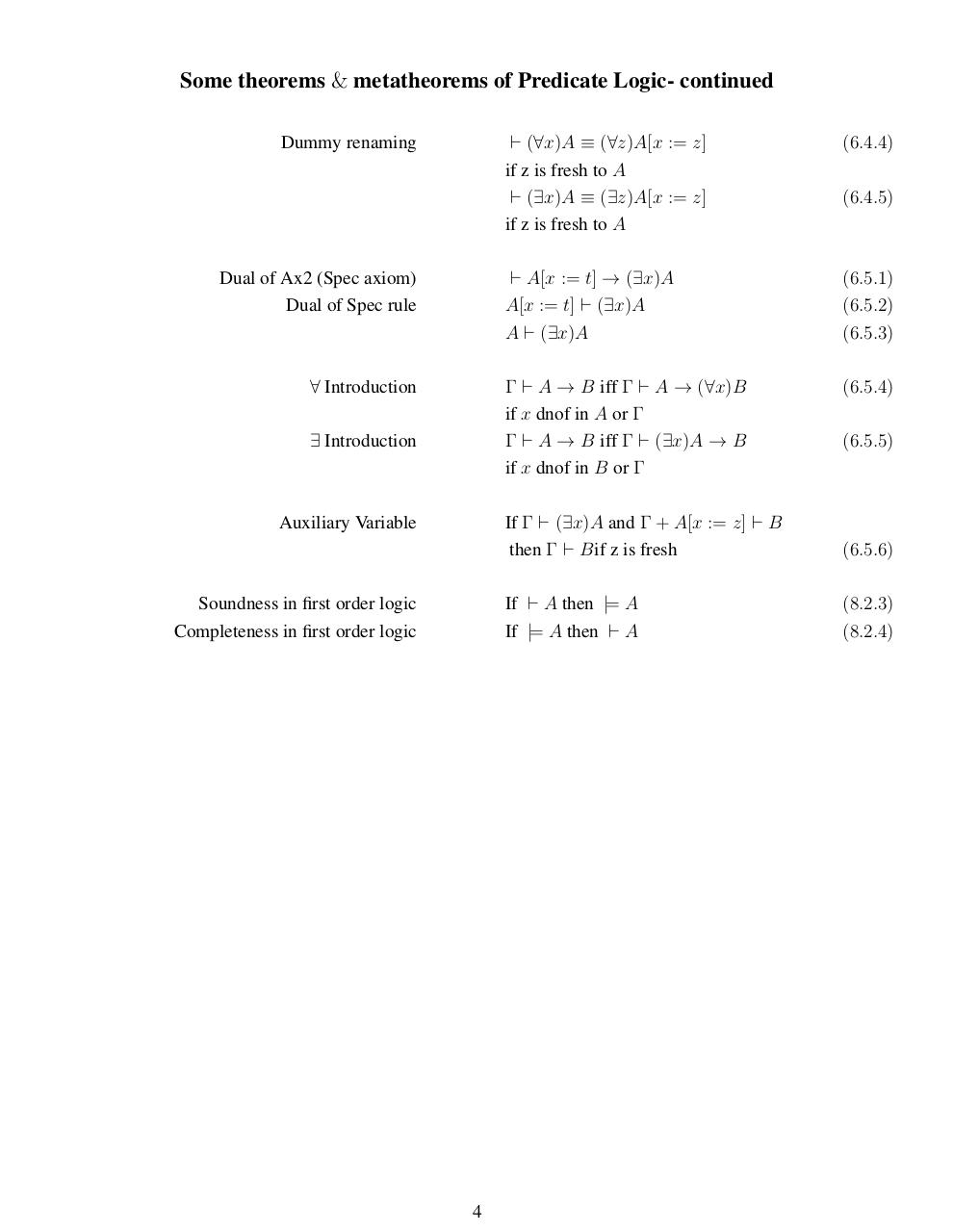# Theorems .pdf

### File information

Original filename: Theorems.pdf

This PDF 1.4 document has been generated by LaTeX with hyperref package / pdfTeX-1.40.10, and has been sent on pdf-archive.com on 07/06/2017 at 00:37, from IP address 89.249.x.x. The current document download page has been viewed 532 times.
File size: 68 KB (4 pages).
Privacy: public file

Theorems.pdf (PDF, 68 KB)

### Document preview

Axioms of Boolean Logic
Associativity of ≡
Symmetry of ≡
&gt;vs. ⊥
introduction of ¬
Associativity of ∨
Symmetry of ∨
Idempotency of ∨
Distributivity of ∨ over ≡
Excluded Middle
Golden Rule
Implication

((A ≡ B) ≡ C) ≡ (A ≡ (B ≡ C))
(A ≡ B) ≡ (B ≡ A)

(1)
(2)

&gt;≡⊥≡⊥
¬A ≡ A ≡ ⊥

(3)
(4)

(A ∨ B) ∨ C ≡ A ∨ (B ∨ C)
A∨B ≡B∨A
A∨A≡A
A ∨ (B ≡ C) ≡ A ∨ B ≡ A ∨ C
A ∨ ¬A

(5)
(6)
(7)
(8)
(9)

A∧B ≡A≡B ≡A∨B
A→B ≡A∨B ≡B

(10)
(11)

Some Theorems of Boolean Logic
Transitivity

Eqn + Leib Merged
Redundant True

Double Negation

de Morgan 1
de Morgan 2

A ≡ B, B ≡ C ` A ≡ C
`A≡A
`&gt;
C[p := A], A ≡ B ` C[p := B]
`&gt;≡A≡A

(1.4.13(c))
(1.4.13(d))
(2.1.15)
(2.1.16)
(2.1.21)

` ¬(A ≡ B) ≡ ¬A ≡ B
` ¬¬A ≡ A
` &gt; ≡ ¬⊥
`A∨&gt;
`A∨⊥≡A

(2.4.1)
(2.4.4)
(2.4.5)
(2.4.7)
(2.4.10)

` A → B ≡ ¬A ∨ B
` ¬A ∨ B ≡ A ∨ B ≡ B
` A → (B ≡ C) ≡ A → B ≡ A → C
` A ∧ B ≡ ¬(¬A ∨ ¬B)
` A ∨ B ≡ ¬(¬A ∧ ¬B)

(2.4.11)
(2.4.12)
(2.4.13)
(2.4.17)
(2.4.18)

`A∧A≡A
`A∧&gt;≡A
`A∧⊥≡⊥

(2.4.19)
(2.4.20)
(2.4.21)

1

Some Theorems of Boolean Logic- continued
Distributivity of ∨ over ∧
Distributivity of ∧ over ∨
Distributivity of → over ∧
Ping-Pong Theorem
Merge

Split
Cut Rule

Modus Ponens
Transitivity of →

Proof by Cases

` A ∨ (B ∧ C) ≡ (A ∨ B) ∧ (A ∨ C)
` A ∧ (B ∨ C) ≡ (A ∧ B) ∨ (A ∧ C)

(2.4.23(i))
(2.4.23(ii))

` A → (B ∧ C) ≡ (A → B) ∧ (A → C)
` (A ∨ B) → C ≡ (A → C) ∧ (B → C)
` A ≡ B ≡ (A → B) ∧ (B → A)

(2.4.25)
(2.4.24)
(2.4.26)

A, B ` A ∧ B
A∨A`A
A`A∨B
A∧B `A

(2.5.1(1))
(2.5.1(2))
(2.5.1(3))
(2.5.1(4))

A ∨ B, ¬A ∨ C ` B ∨ C
A ∨ B, ¬A ∨ B ` B
A ∨ B, ¬A ` B
A, ¬A ` ⊥

(2.5.4)
(2.5.5)
(2.5.6)
(2.5.7)

A, A → B ` B
A → B, B → C ` A → C

(2.5.3)
(2.5.9)

A → C, B → D ` A ∨ B → C ∨ D
A → C, B → C ` A ∨ B → C
A → C, ¬A → C ` C

(2.5.10)
(2.5.11)
(2.5.13)

2

Axioms of Predicate Logic
The axioms of Predicate Logic consist of all partial generalizations of the following schemata:
Ax1
Ax2
Ax3
Ax4
Ax5
Ax6

All tautologies.
(∀x)A → A[x := t]
(∀x)(A → B) → (∀x)A → (∀x)B
A → (∀x)A, if x dnof in A
x=x
s = t → (A[x := s] ≡ A[x := t])

Specialization axiom

Identity axiom
Leibniz axiom for equality

Some theorems &amp; metatheorems of Predicate Logic
Weak Generalization

Specialization

Distributivity of ∀ over ∧
∀-monotonicity

Weak Leibniz
Strong Leibniz

If Γ ` A, then Γ ` (∀x)A
if x dnof in any formula of Γ
If ` A, then ` (∀x)A

(6.1.3)

(∀x)A ` A[x := t]
(∀x)A ` A

(6.1.5)
(6.1.6)

` (∀x)(∀y)A ≡ (∀y)(∀x)A
` (∀x)(A ∧ B) ≡ (∀x)A ∧ (∀x)B

(6.1.8)
(6.1.7)

If Γ ` A → B, then Γ ` (∀x)A → (∀x)B
if x dnof in any formula of Γ

(6.1.9)

If Γ ` A ≡ B, then Γ ` (∀x)A ≡ (∀x)B
if x dnof in any formula of Γ

(6.1.11)

If ` A ≡ B, then ` C[p\A] ≡ C[p\B]
A ≡ B ` C[p := A] ≡ C[p := B]

(6.2.1)
(6.2.3)

` (∀x)(A → B) ≡ A → (∀x)B
if x dnof inA

(6.4.1)

` (∀x)(A ∨ B) ≡ A ∨ (∀x)B
if x dnof inA

(6.4.2)

` (∃x)(A ∧ B) ≡ A ∧ (∃x)B
if x dnof inA

(6.4.3)

3

(6.1.1)

Some theorems &amp; metatheorems of Predicate Logic- continued
Dummy renaming

Dual of Ax2 (Spec axiom)
Dual of Spec rule

∀ Introduction
∃ Introduction

Auxiliary Variable

Soundness in first order logic
Completeness in first order logic

4

` (∀x)A ≡ (∀z)A[x := z]
if z is fresh to A
` (∃x)A ≡ (∃z)A[x := z]
if z is fresh to A

(6.4.4)

` A[x := t] → (∃x)A
A[x := t] ` (∃x)A
A ` (∃x)A

(6.5.1)
(6.5.2)
(6.5.3)

Γ ` A → B iff Γ ` A → (∀x)B
if x dnof in A or Γ
Γ ` A → B iff Γ ` (∃x)A → B
if x dnof in B or Γ

(6.5.4)

(6.4.5)

(6.5.5)

If Γ ` (∃x)A and Γ + A[x := z] ` B
then Γ ` Bif z is fresh

(6.5.6)

If ` A then |= A
If |= A then ` A

(8.2.3)
(8.2.4)#### HTML Code

Copy the following HTML code to share your document on a Website or Blog

#### QR Code### Related keywords